# Metric geometry : Investment beam circumferencesBy studying the Investment in the plane We analyze the transformation of the basic geometric elements (straight and girth) Two different cases, when the investment center was on them or at any point that was not theirs.

Transformation through investment in geometric shapes grouped elements can be of interest to use the investment as a tool for analysis in complex problems. In this case study transforming “beams circumferences corradicales” through various investments that transform. Later these transformations need to solve the problem “Apolonio” (circumference with three tangency constraints) o la “Generalization of the problem of Apollonius” (circumferences with three angular restrictions).

### Transformation circumference investment

If we consider that a line can be a circle of infinite radius, generalizing, We conclude that in any case a circle is transformed by an investment in another circle.

We saw that both circumferences were also related by homotecia the investment center. Figure I is the center point of a positive investment that transforms the circumference c on the circumference c’. Investment power is represented by Ck self-inverting circumference whose radius is the root of this power. Points A and A’ are inverse elements but are not homothetic while tangency from the center investment, T and T’, They are the ones who are to reverse time and homothetic center point I.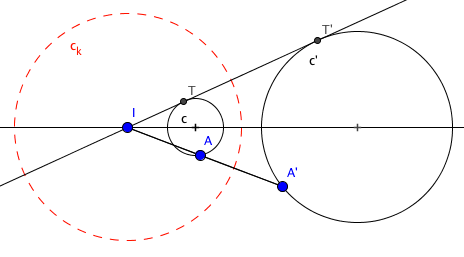At the time we saw the reverse circumferences centers are aligned with the center of investment and, although they are homothetic in homotecias relating to the circumferences, They are not inverse in investment.

### Parabolic Beam investment.

Los beams circumferences parabolic corradicales are formed by those circumferences are tangent to each other at one point. This point belongs to radical axis all of them and has no power regarding any circumference of the beam to belong to all of them.If we consider the circles tangent at the point “A” the circumference c we have invested before, their transforms pass through point A’ transformed the A and as cut only at that point to the circumference c (his tangent) only cut in the transformed A’ the circumference c’ reverse so determined shall be tangents and again a parabolic beam.The circumference of the original beam passing through the investment center will become a tangent to the beam transformed into point A’ and, therefore, in its radical axis.

By investing with the investment center point common to all transformed will be a set of parallel lines each other and perpendicular to the base line of the original beam, as the inverse of a circle containing the investment center is a straight direction normal to the diameter of the circumference passing through the center investment.### Make investment Elíptico

Los corradicales beam circumferences elliptical type They are formed by those circumferences passing through two common points to the whole beam. These dots called “key points of the beam” they belong to radical axis beam power and have no respect any circumference of the beam to belong to all of them.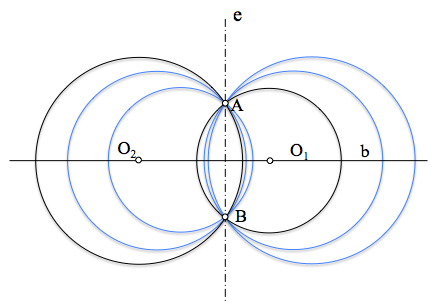Analogous to what we have seen for the parabolic case, passing through two points all the circumferences of their investment transformed beam pass through the two inverse points of the above and consequently will determine a new elliptical beam circumferences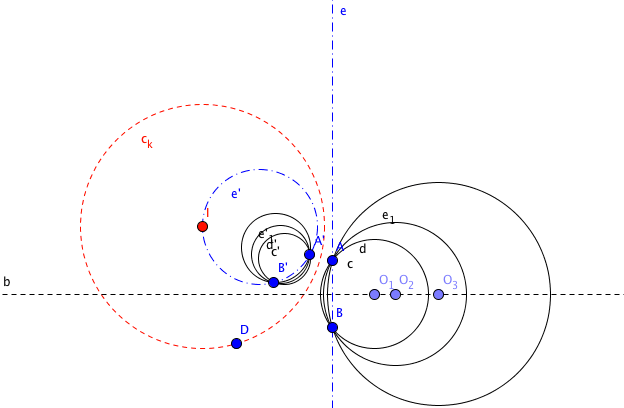The radical axis and the beam will become a circle and’ which passes through the center investment.

By investing investment center one of the points common to all of them (fundamental points) transformed will be a set of straight lines that pass through the inverse of the other common point which is not investment center.

We see therefore that transform into a bundle of straight.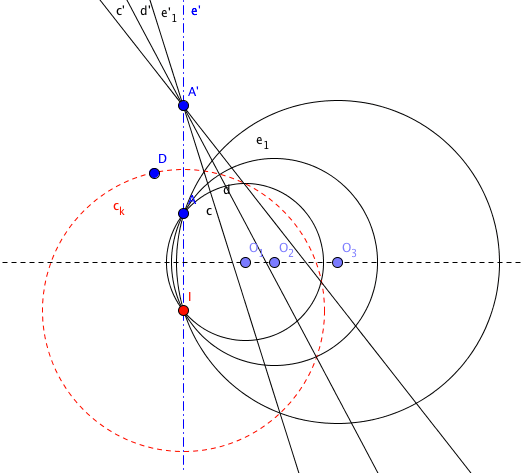### Make investment Hyperbolic

Los corradicales beam circumferences of hyperbolic type They are formed by those circles who have no common point, their centers lie on a straight (Based beam) and have a radical axis common. Circumferences smaller diameter have zero radius (colon, L1 and L2) denominating “limits the beam points“.By studying these beams saw the limits of hyperbolic beam points were the key points of the elliptical beam conjugate.This relationship between the hyperbolic and elliptical beams allows us to deduce that a beam hyperbolic to be transformed by an investment turns into another beam hyperbolic points whose boundaries are transformed points limits original beam.

Indeed, if we transform the two limit points will become two new inverse points of the above. The elliptical beam passing through them will become an elliptical beam to pass through the transformed and, as the transformation is as (maintains the angles), hyperbolic beam which is coupled to the elliptical, will be transformed into a new beam should be orthogonal to the inverse of the elliptical.

Of special interest is the investment limits one of the center points.

The figure represented a beam corradical hyperbolic points with limits L1 and L2, whose circumferences are centered on the base line b, share the radical axis and y are orthogonal to the circumference of the center beam orthogonal beam (intersection of the base line and the radical axis). This circle will pass through points limits.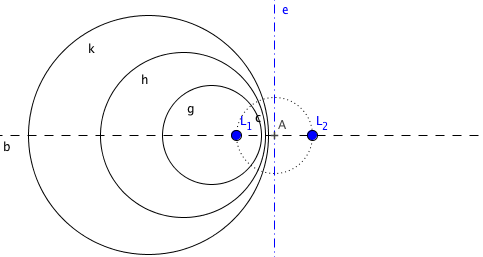If we take as an investment center of the points limits, for example L2, with any power, the other boundary point will become a new point, L1′, and the circle passing through the points limits, L1 and L2, Hyperbolic belonging to conjugate beam beam is transformed into a line that will pass through L1′ inverse L1.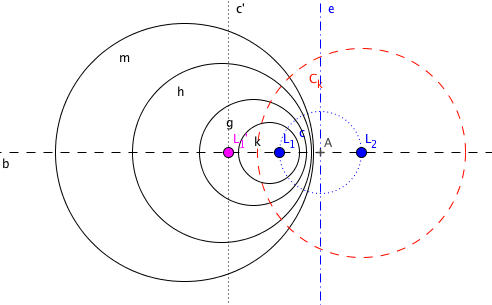Like all the hyperbolic beam circumferences are orthogonal to this circle, their inverses it will reverse it straight, so you have to have your heart in this straight.

Moreover as the centers of the new reverse circumferences must be aligned with the centers of the original circumferences and investment center, being the investment center in the straight base, the new reverse circumferences of the beam should be centered on the base line.

Namely, reverse circumferences must have their center at the base line and the line transform of the circle through L1 and L2. The intersection of these lines is the L1 point′ inverse L1, por lo que el haz de circunferencias se convierte en un conjunto de circunferencias concéntricas.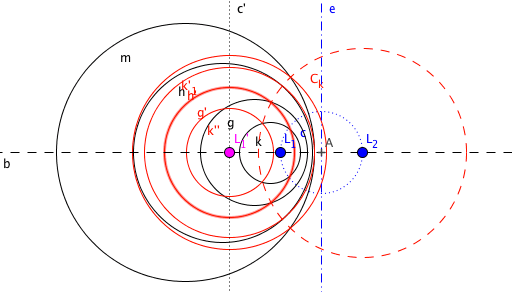Veremos el interés de estas transformaciones al aplicarlas en la resolución de problemas de tangencias o angularidad.

### Otras inversiones de interés.

Cabe destacar en todos los casos, haces elípticos, parabolic and hyperbolic, el interés que tiene usar un punto del eje radical como centro de inversión y potencia la de este punto respecto de las circunferencias del haz. En este caso el haz se transforma en sí mismo. Se deja al lector el análisis gráfico de este interesante caso.